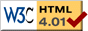# sims.roller.BrachistoApp

previous next
• Sim
• Graph
• Time Graph
• Multi Graph

Test macros for x1, x2, y1, y2 whereas these should not be changed: x_1, x_2, y_1, y_2. Here is an inline version: y2 = y1 + f1(x1) + f2(x2) of a math equation. And again these should not be changed: x_1, x_2, y_1, y_2.

Here is an example of using the eqn_start macro:

y2 = y1 + f1(x1) + f2(x2)

Here is a MathJax version $$y_1 + f1(x_1) + f2(x_2)$$ which is inline within text.

## The Euler-Lagrange Equation

The Euler-Lagrange equation is a differential equation that $$y(x)$$ must satisfy in order to minimize (or possibly maximize) an integral of the form $$I = \int_{x_1}^{x_2} f(x, y, y') dx$$ where also $$y(x)$$ must pass through the two fixed end points $$y(x_1) = y_1$$ and $$y(x_2) = y_2$$. Through reasoning about families of curves that include the minimizing curve $$y(x)$$, we can get the Euler-Lagrange equation: $$\frac{\partial f}{\partial y} - \frac{d}{dx} \left( \frac{\partial f}{\partial y'} \right) = 0$$

This page is for testing and development please see myPhysicsLab for the published version of this simulation.